Learning Library

Easter Math: Addition and Subtraction

Spring into the season with this collection of kindergarten Easter addition and subtraction worksheets. Your child will master basic math building blocks with these egg-cellent printables.

Click on a worksheet in the set below to see more info or download the PDF.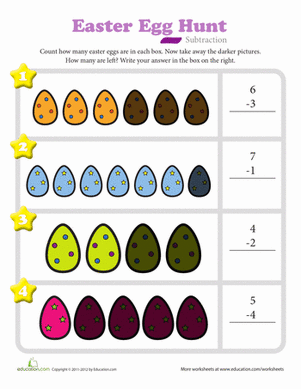Easter Egg Hunt Subtraction

Introduce your kindergartener to subtraction. Count the total number of eggs in each box. Then count the number of dark eggs and take that number away.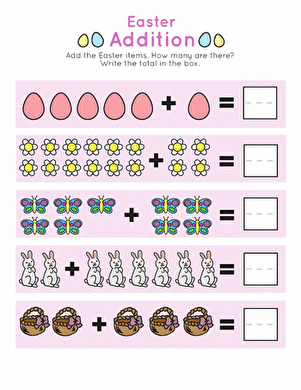Celebrate spring with some Easter addition practice. This roundup of picture equations provides a great visual intro to addition.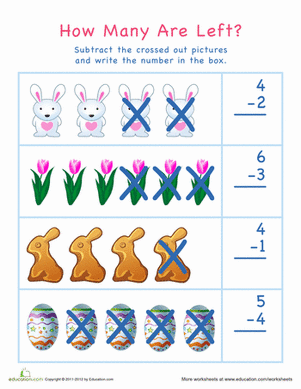Easter Chocolate Subtraction: How Many Are Left?

Your child will count and subtract in this Easter-themed worksheet, perfect for visual learners. Help your kid see how many bunnies, tulips, and eggs are left.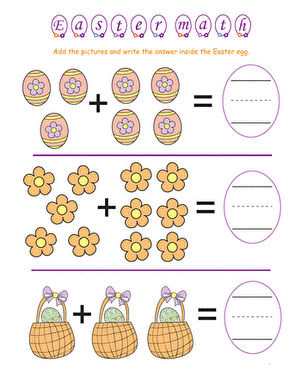Add up simple addition problems using Easter themed visual aids, such as Easter eggs and flowers. Try our Easter addition worksheet with your child this spring.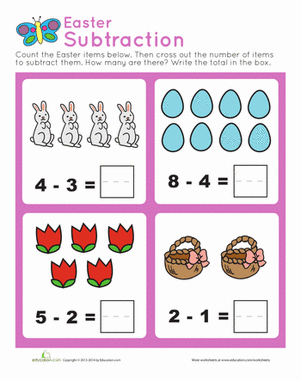Easter Subtraction Practice

Here's a colorful set of drill sheets for kindergarteners who are ready to learn about subtraction.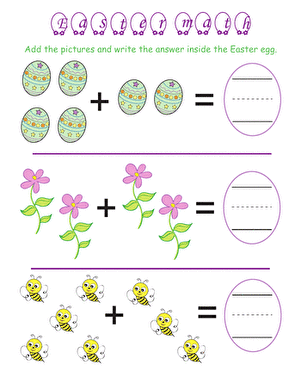Easter Math: Busy Bee Addition

Help your busy bee get some addition practice in on Easter with this addition worksheet. She'll use visual clues to help her tally up addition problems.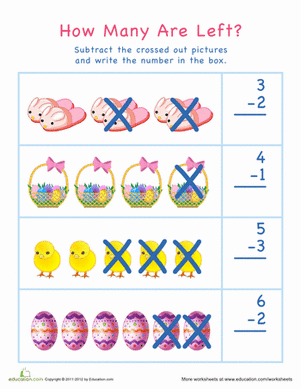Easter Egg Subtraction: How Many Are Left?

Kids will use subtraction and counting skills with our Easter math worksheet. Kids who use counters or pictures during math practice will love this worksheet.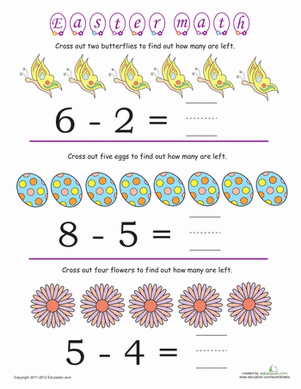Easter Math: Butterfly Subtraction

Your child will use Easter symbols as visual aids in this Easter subtraction worksheet. Try this Easter subtraction worksheet with your little bunny.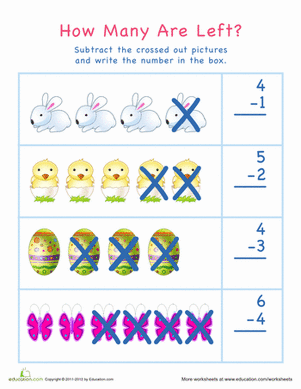Easter Bunny Math

Help your kid practice subtraction and counting skills with this Easter math worksheet. This Easter-themed worksheet is perfect for visual learners especially.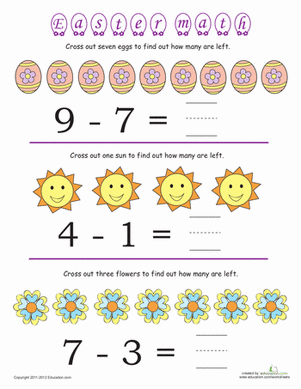Easter Math #4: Subtraction

Help your young mathematician get some subtraction practice in on Easter with this worksheet. Use Easter eggs and flowers to help your child subtract.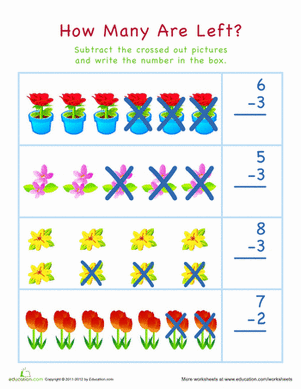How Many Flowers Are Left?

Visual learners will learn subtraction basics with our colorful, flower-themed worksheet. Kids will count picture of flowers, then cross out images to subtract.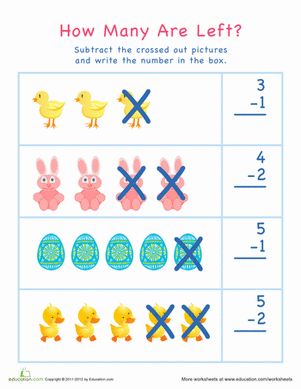Easter Subtraction: How Many Are Left?

Counting and subtraction come together in this Easter math worksheet for visual learners. Kids will learn how to subtract by ones and twos in this worksheet.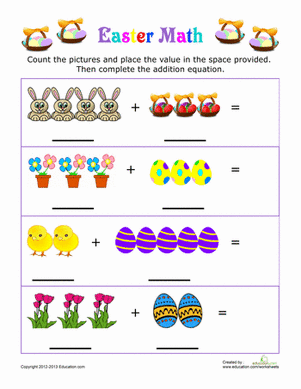Who's that coming down the path? Why, it must be the Easter bunny! Give your first grader a fun way to practice her basic addition.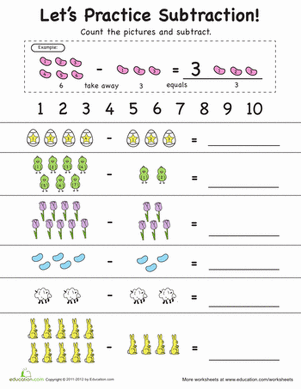Subtraction Practice: Spring Into It!

This subtraction practice worksheet combines number writing with pictures and counting to make beginning subtraction easier for your child!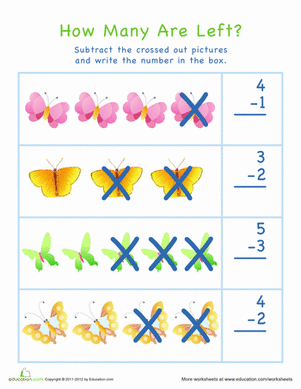How Many Butterflies Are Left?

Cross out butterflies to learn subtraction basics in this math worksheet. Kids will solve subtraction problems by crossing out pictures of butterflies.

Want to download this whole set as a single PDF?

Create new collection

0

New Collection>

0 items

What could we do to improve Education.com?

Please note: Use the Contact Us link at the bottom of our website for account-specific questions or issues.

What would make you love Education.com?